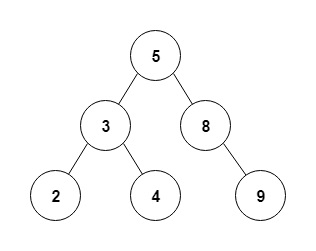# C++ Program to Perform Preorder Non-Recursive Traversal of a Given Binary Tree

Tree traversal is a form of graph traversal. It involves checking or printing each node in the tree exactly once. The preorder traversal of a binary search tree involves visiting each of the nodes in the tree in the order (Root, Left, Right).

An example of Preorder traversal of a binary tree is as follows.

A binary tree is given as follows.Preorder Traversal is: 5 3 2 4 8 9

A program to perform preorder non-recursive traversal is given as follows.

## Example

Live Demo

#include<iostream>
#include <stack>
using namespace std;
struct node {
int data;
struct node *left;
struct node *right;
};
struct node *createNode(int val) {
struct node *temp = (struct node *)malloc(sizeof(struct node));
temp→data = val;
temp→left = temp→right = NULL;
return temp;
}
void preorder(struct node *root) {
if (root == NULL)
return;
stack<node *> nodeStack;
nodeStack.push(root);
while (nodeStack.empty() == false) {
struct node *temp_node = nodeStack.top();
cout<< temp_node->data <<" ";
nodeStack.pop();
if (temp_node→right)
nodeStack.push(temp_node->right);
if (temp_node→left)
nodeStack.push(temp_node->left);
}
}
struct node* insertNode(struct node* node, int val) {
if (node == NULL) return createNode(val);
if (val < node→data)
node→left = insertNode(node→left, val);
else if (val > node→data)
node→right = insertNode(node→right, val);
return node;
}
int main() {
struct node *root = NULL;
root = insertNode(root, 5);
insertNode(root, 8);
insertNode(root, 3);
insertNode(root, 2);
insertNode(root, 6);
insertNode(root, 9);
insertNode(root, 4);
cout<<"Pre-Order traversal of the Binary Search Tree is: ";
preorder(root);
}

## Output

Pre-Order traversal of the Binary Search Tree is: 5 3 2 4 8 6 9

In the above program, the structure node creates the node of a tree. This structure is a self referential structure as it contains pointers of struct node type. This structure is shown as follows.

struct node {
int data;
struct node *left;
struct node *right;
};

The function createNode() creates a node temp and allocates it memory using malloc. The data value val is stored in data of temp. NULL is stored in left and right pointers of temp. This is demonstrated by the following code snippet.

struct node *createNode(int val) {
struct node *temp = (struct node *)malloc(sizeof(struct node));
temp→data = val;
temp→left = temp→right = NULL;
return temp;
}

The function preorder() uses a stack to print the elements of the tree in preorder. First the root node is inserted into the stack. A while loop is started that runs until the stack is not empty. First the data in the topnode element of the stack is displayed and then, the node is popped. Then the right and left nodes of the above node are pushed into the stack.

This function is demonstrated using the following code snippet.

void preorder(struct node *root) {
if (root == NULL)
return;
stack<node *> nodeStack;
nodeStack.push(root);
while (nodeStack.empty() == false) {
struct node *temp_node = nodeStack.top();
cout<< temp_node->data <<" ";
nodeStack.pop();
if (temp_node→right)
nodeStack.push(temp_node→right);
if (temp_node→left)
nodeStack.push(temp_node→left);
}
}

The function insertNode() inserts the required value in the binary tree at its correct position. If the node is NULL, then createNode is called. Otherwise, the correct position for the node is found in the tree. This can be observed in the following code snippet.

struct node* insertNode(struct node* node, int val) {
if (node == NULL) return createNode(val);
if (val < node→data)
node→left = insertNode(node→left, val);
else if (val > node→data)
node->right = insertNode(node→right, val);
return node;
}

In the main() function, the root node is first defined as NULL. Then all the nodes with the required values are inserted into the binary search tree. This is shown below.

struct node *root = NULL;
root = insertNode(root, 5);
insertNode(root, 8);
insertNode(root, 3);
insertNode(root, 2);
insertNode(root, 6);
insertNode(root, 9);
insertNode(root, 4);

Finally, the function preorder() is called using the root node of the tree and all the tree values are displayed in preorder. This is given below.

cout<<"Pre-Order traversal of the Binary Search Tree is: ";
preorder(root);